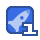# MonteCarlo 算法35收藏

Generalized wiener process △x=a△t+bE△t where a and b are constants Ax is normally distributed with mean of△x=△t standard deviation of△x=by△t variance of△x=b2△t For Long Period change in value of x in any interval is normally distributed with mean of change in x=aT standard deviation of change in x=vT variance of change in x=b T Ito process dx=a(r, tdt +b(x, t)dz where a and b are functions of the underlying variable, x, and time,t Ito's lemma A function G of x and t follow the process dgdG 1 dG dg dG=a+ b- dt+obd2 dx dt 2 d dx where dz is a wiener process G follows an Ito process with drift rate dg dg 1 dG b X at 2 d and a variance rate of dG b dx 8 Application to In(Stock Price) Stock Price model ds=uSdt +osd G=In s Since dG 1 dg 1 dG =0 ds s ds s dt the process follow by g is 2 O dG=l u 十O 2 Property Change G between current time, t, and some future time T is normallly distributed with mean and variance 10 Simulation model △f+OE S(t+△t)=S(t)e Where e is drawn from a standard normal distribution Generating Random Samples of Distribution Froy Xis a random variable with uniform distribution Generate samples of y with distribution Fry) Y=FY(X) Fy(x) P(Y≤y) P(X≤F(y Fy(y X is uniform distributed F(y) BoX Muller generating two independent standard normals from two independent uniforms no closed form for N(=P(X sx) e √2z a cannot not apply Y=FY(X) 3 Some mathematics X三 2 e dx e C polar coordinates x=rcos 0 y=rsin 6, dxdy= rdrde 2丌 e2rrd6=2丌 丌 0 0 Probabilistic Interpretation C yis a pair of independent standard normals probability density r+ (X,Y) (x,y)= 2兀 radially symmetric polar coordinates(R, 6) B is uniformly distributed in the interval [o, 2I Rhas a distribution function P(RSr=I e 2 udud0= e 2 udu=1 10J02x Box Muller R=F(X)=y-2n(1-X If X is standard uniform, R has distribution of Fr(r) Wecan also replace(1-x) U, where U is standard uniform R 2Inv Summary Box Muller takes 2 independent standard uniform variables u, and u generates 2 independent normals X andY 8=2U,R=-2lnU2, X=Rcos e, Y=Rsin 0 Polar Rejection Method 1. Set V=20-l and V,=202 2. Compute W=V+V, 3. If W>l, return to step 1. Otherwise, set (-2lnW), 2In w v W W 2 17 Relating Box Muller and Polar rejection V, and v, are independent uniform W=V+v2 (vv2) P(W≤w=P(V12+V2)≤w) ∴ W is uniforn R=√2lnW cos 6= V, sIn 6

...展开详情

• 1
资源
• 0
粉丝
•等级MonteCarlo 算法 22积分/C币 立即下载
1/922积分/C币 立即下载